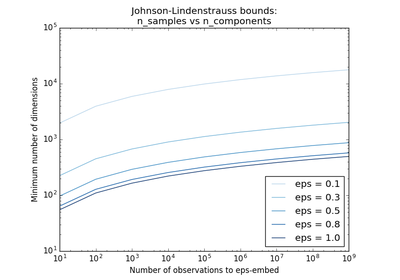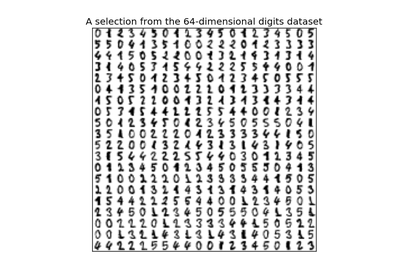# `sklearn.random_projection`.SparseRandomProjection¶

class `sklearn.random_projection.``SparseRandomProjection`(n_components='auto', density='auto', eps=0.1, dense_output=False, random_state=None)[source]

Reduce dimensionality through sparse random projection

Sparse random matrix is an alternative to dense random projection matrix that guarantees similar embedding quality while being much more memory efficient and allowing faster computation of the projected data.

If we note s = 1 / density the components of the random matrix are drawn from:

• -sqrt(s) / sqrt(n_components) with probability 1 / 2s
• 0 with probability 1 - 1 / s
• +sqrt(s) / sqrt(n_components) with probability 1 / 2s

Read more in the User Guide.

Parameters: n_components : int or ‘auto’, optional (default = ‘auto’) Dimensionality of the target projection space. n_components can be automatically adjusted according to the number of samples in the dataset and the bound given by the Johnson-Lindenstrauss lemma. In that case the quality of the embedding is controlled by the `eps` parameter. It should be noted that Johnson-Lindenstrauss lemma can yield very conservative estimated of the required number of components as it makes no assumption on the structure of the dataset. density : float in range ]0, 1], optional (default=’auto’) Ratio of non-zero component in the random projection matrix. If density = ‘auto’, the value is set to the minimum density as recommended by Ping Li et al.: 1 / sqrt(n_features). Use density = 1 / 3.0 if you want to reproduce the results from Achlioptas, 2001. eps : strictly positive float, optional, (default=0.1) Parameter to control the quality of the embedding according to the Johnson-Lindenstrauss lemma when n_components is set to ‘auto’. Smaller values lead to better embedding and higher number of dimensions (n_components) in the target projection space. dense_output : boolean, optional (default=False) If True, ensure that the output of the random projection is a dense numpy array even if the input and random projection matrix are both sparse. In practice, if the number of components is small the number of zero components in the projected data will be very small and it will be more CPU and memory efficient to use a dense representation. If False, the projected data uses a sparse representation if the input is sparse. random_state : integer, RandomState instance or None (default=None) Control the pseudo random number generator used to generate the matrix at fit time. n_component_ : int Concrete number of components computed when n_components=”auto”. components_ : CSR matrix with shape [n_components, n_features] Random matrix used for the projection. density_ : float in range 0.0 - 1.0 Concrete density computed from when density = “auto”.

References

 [R238] Ping Li, T. Hastie and K. W. Church, 2006, “Very Sparse Random Projections”. http://web.stanford.edu/~hastie/Papers/Ping/KDD06_rp.pdf
 [R239] D. Achlioptas, 2001, “Database-friendly random projections”, https://users.soe.ucsc.edu/~optas/papers/jl.pdf

Methods

 `fit`(X[, y]) Generate a sparse random projection matrix `fit_transform`(X[, y]) Fit to data, then transform it. `get_params`([deep]) Get parameters for this estimator. `set_params`(\*\*params) Set the parameters of this estimator. `transform`(X[, y]) Project the data by using matrix product with the random matrix
`__init__`(n_components='auto', density='auto', eps=0.1, dense_output=False, random_state=None)[source]
`fit`(X, y=None)[source]

Generate a sparse random projection matrix

Parameters: X : numpy array or scipy.sparse of shape [n_samples, n_features] Training set: only the shape is used to find optimal random matrix dimensions based on the theory referenced in the afore mentioned papers. y : is not used: placeholder to allow for usage in a Pipeline. self :
`fit_transform`(X, y=None, **fit_params)[source]

Fit to data, then transform it.

Fits transformer to X and y with optional parameters fit_params and returns a transformed version of X.

Parameters: X : numpy array of shape [n_samples, n_features] Training set. y : numpy array of shape [n_samples] Target values. X_new : numpy array of shape [n_samples, n_features_new] Transformed array.
`get_params`(deep=True)[source]

Get parameters for this estimator.

Parameters: deep : boolean, optional If True, will return the parameters for this estimator and contained subobjects that are estimators. params : mapping of string to any Parameter names mapped to their values.
`set_params`(**params)[source]

Set the parameters of this estimator.

The method works on simple estimators as well as on nested objects (such as pipelines). The latter have parameters of the form `<component>__<parameter>` so that it’s possible to update each component of a nested object.

Returns: self :
`transform`(X, y=None)[source]

Project the data by using matrix product with the random matrix

Parameters: X : numpy array or scipy.sparse of shape [n_samples, n_features] The input data to project into a smaller dimensional space. y : is not used: placeholder to allow for usage in a Pipeline. X_new : numpy array or scipy sparse of shape [n_samples, n_components] Projected array.

## Examples using `sklearn.random_projection.SparseRandomProjection`¶The Johnson-Lindenstrauss bound for embedding with random projectionsManifold learning on handwritten digits: Locally Linear Embedding, Isomap...